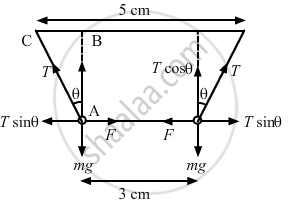Advertisement Remove all ads

# Two Identical Pith Balls Are Charged by Rubbing One Against Other.They Are Suspended from a Horizontal Rod Through Two Strings of Length 20 Cm Each, Separation Between the Suspension Points Being 5 Cm - Physics

Short Note

Two identical pith balls are charged by rubbing one against the other. They are suspended from a horizontal rod through two strings of length 20 cm each, the separation between the suspension points being 5 cm. In equilibrium, the separation between the balls is 3 cm. Find the mass of each ball and the tension in the strings. The charge on each ball has a magnitude 2.0 × 10−8 C.

Advertisement Remove all ads

#### SolutionLet the tension in the string be T and the force of attraction between the two balls be F.
From the free-body diagram of the balls, we get
Tcosθ = mg    ...(1)
Tsinθ = F    ...(2)
From ∆ABC,

$\sin\theta = \frac{1}{20}$

$\cos\theta = \sqrt{1 - \left( \frac{1}{20} \right)^2}$

From equation (1) and (2),

$\tan\theta = \frac{F}{\text{mg}}$

$\therefore \text{m} = \frac{F}{g\tan\theta}$

$\Rightarrow m = \left( \frac{1}{4\pi \epsilon_0}\frac{q^2}{r^2} \right) \times \left( \frac{1}{g\tan\theta} \right)$

$\Rightarrow m = \frac{9 \times {10}^9 \times \left( 2 \times {10}^{- 8} \right)^2}{9 \times {10}^{- 4} \times 0 . 98 \times \tan\theta}$

$\Rightarrow m = 0 . 0082 \text{ kg = 8 . 2 g}$

$T = \frac{F}{\sin\theta} = \frac{9 \times {10}^9 \times \left( 2 \times {10}^{- 8} \right)^2}{9 \times {10}^{- 4} \times \frac{1}{20}}$

$\Rightarrow T = 8 . 2 \times {10}^2$N

Is there an error in this question or solution?
Advertisement Remove all ads

#### APPEARS IN

HC Verma Class 11, Class 12 Concepts of Physics Vol. 2
Chapter 7 Electric Field and Potential
Q 23 | Page 121
Advertisement Remove all ads

#### Video TutorialsVIEW ALL 

Advertisement Remove all ads
Share
Notifications

View all notifications

Forgot password?